# Selina Solutions Concise Mathematics Class 6 Chapter 9: Playing With Numbers

Selina Solutions Concise Mathematics Class 6 Chapter 9 Playing With Numbers provides students a step by step method of solving problems, along with illustrations. Students gain a better idea of concepts covered, by solving tricky questions of the textbook thoroughly. Experts at BYJU’S have solved the questions keeping in mind the understanding capacity of students. Students boost their problem solving skills by referring to these Solutions, provided in simple language.

Some of the concepts discussed in this chapter are interchanging the digits of a number, the generalized form of numbers, tests of divisibility, etc. in a precise and lucid manner. By practising Selina Solutions, students not only clear their doubts but also secure high marks in the annual examinations. Students are advised to download the PDF of Selina Solutions Concise Mathematics Class 6 Chapter 9 Playing With Numbers, from the links given here

## Selina Solutions Concise Mathematics Class 6 Chapter 9: Playing With Numbers Download PDF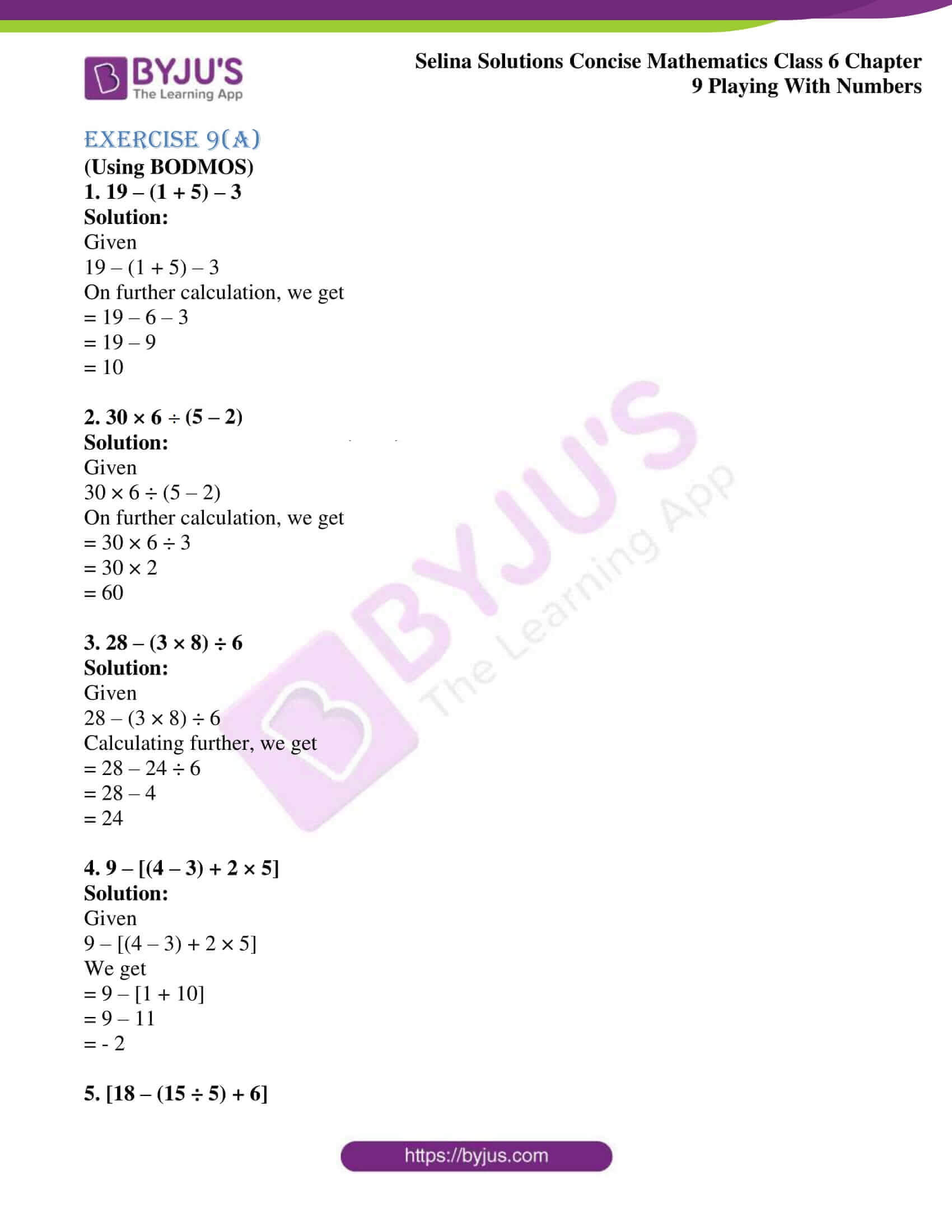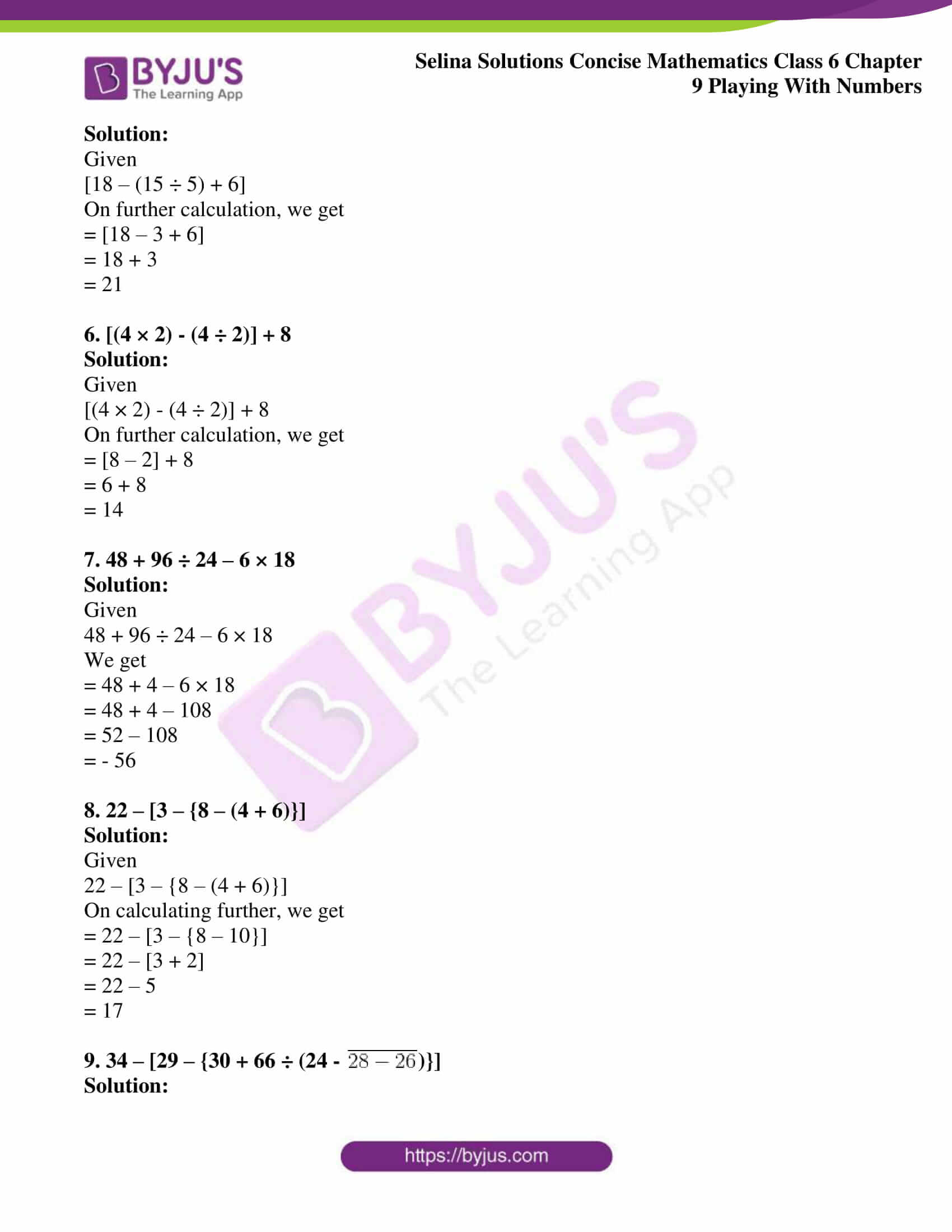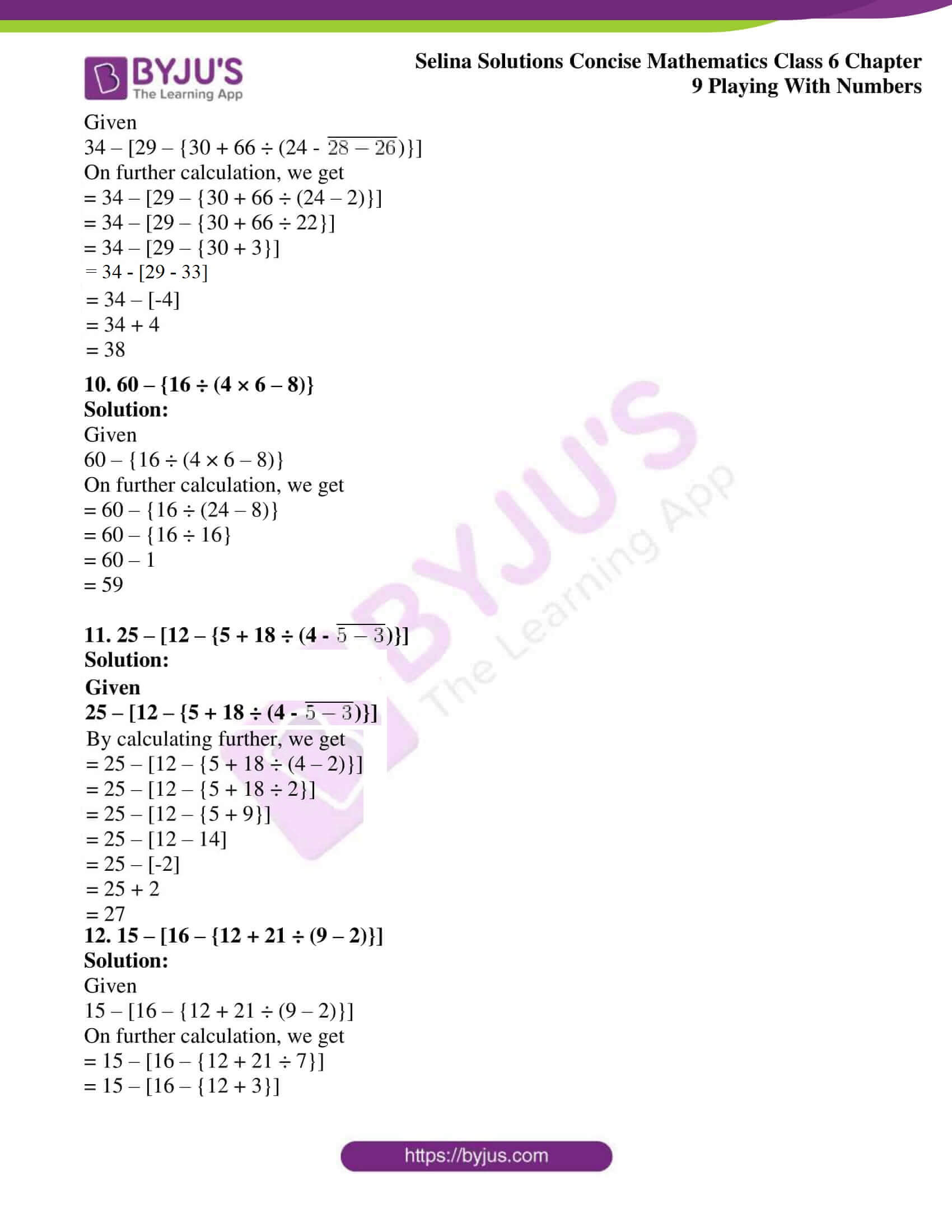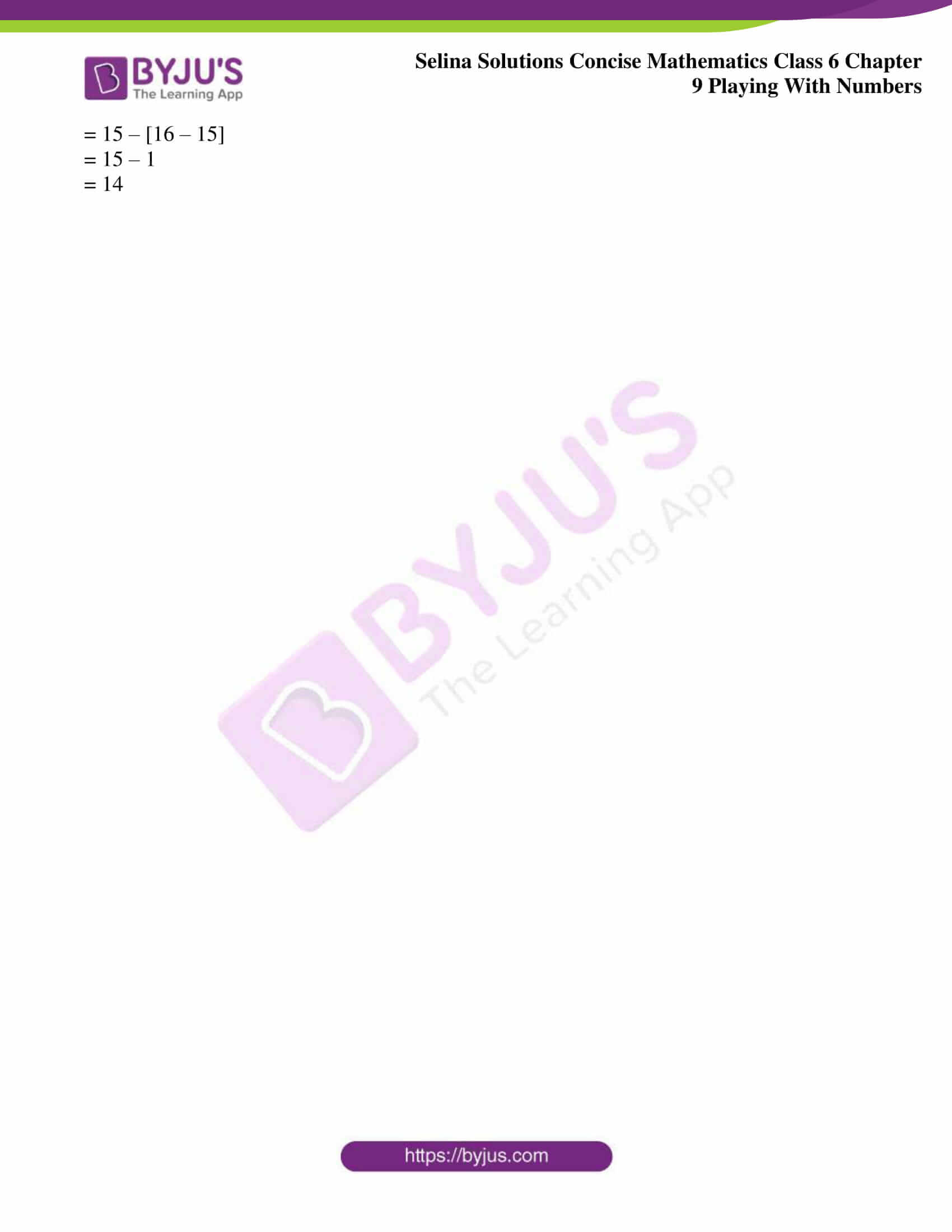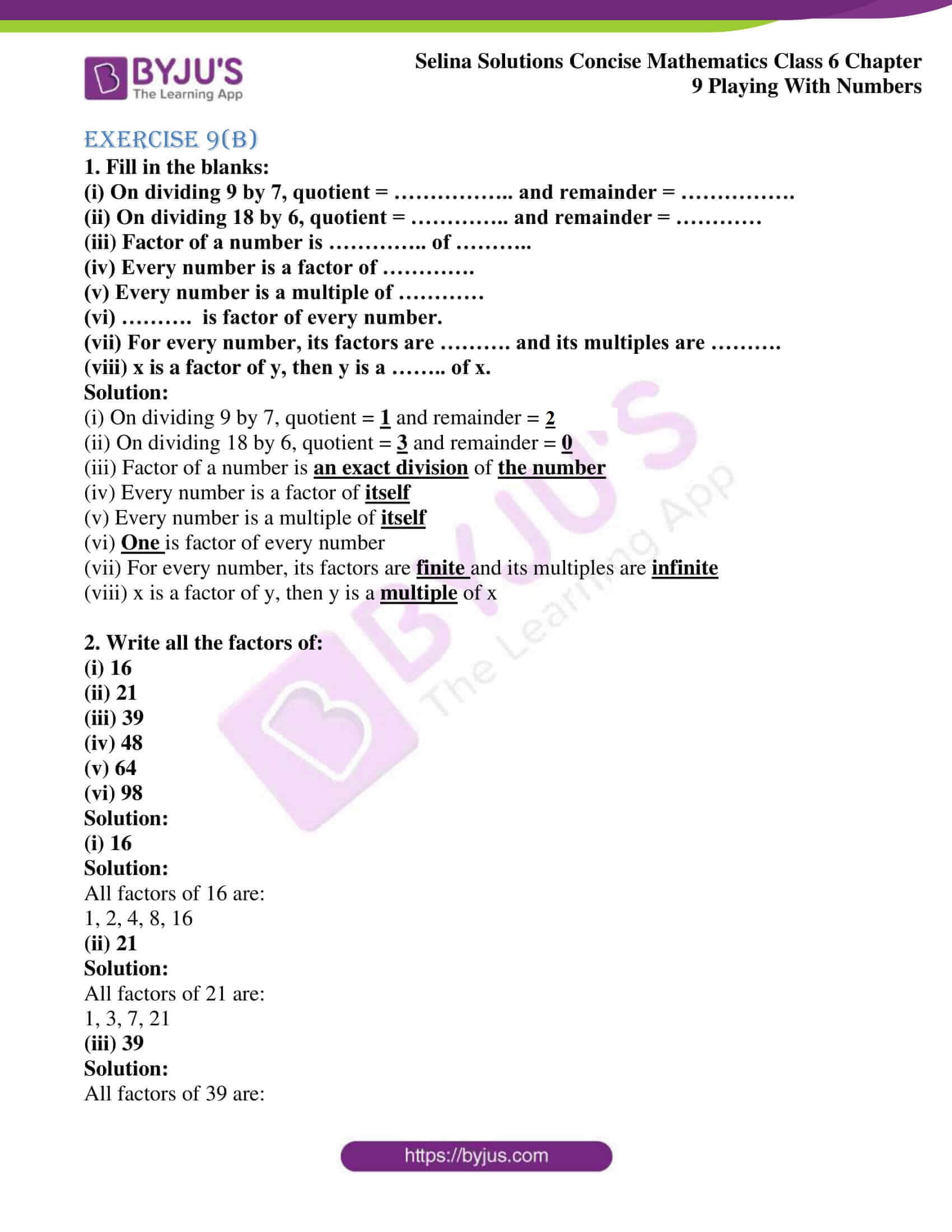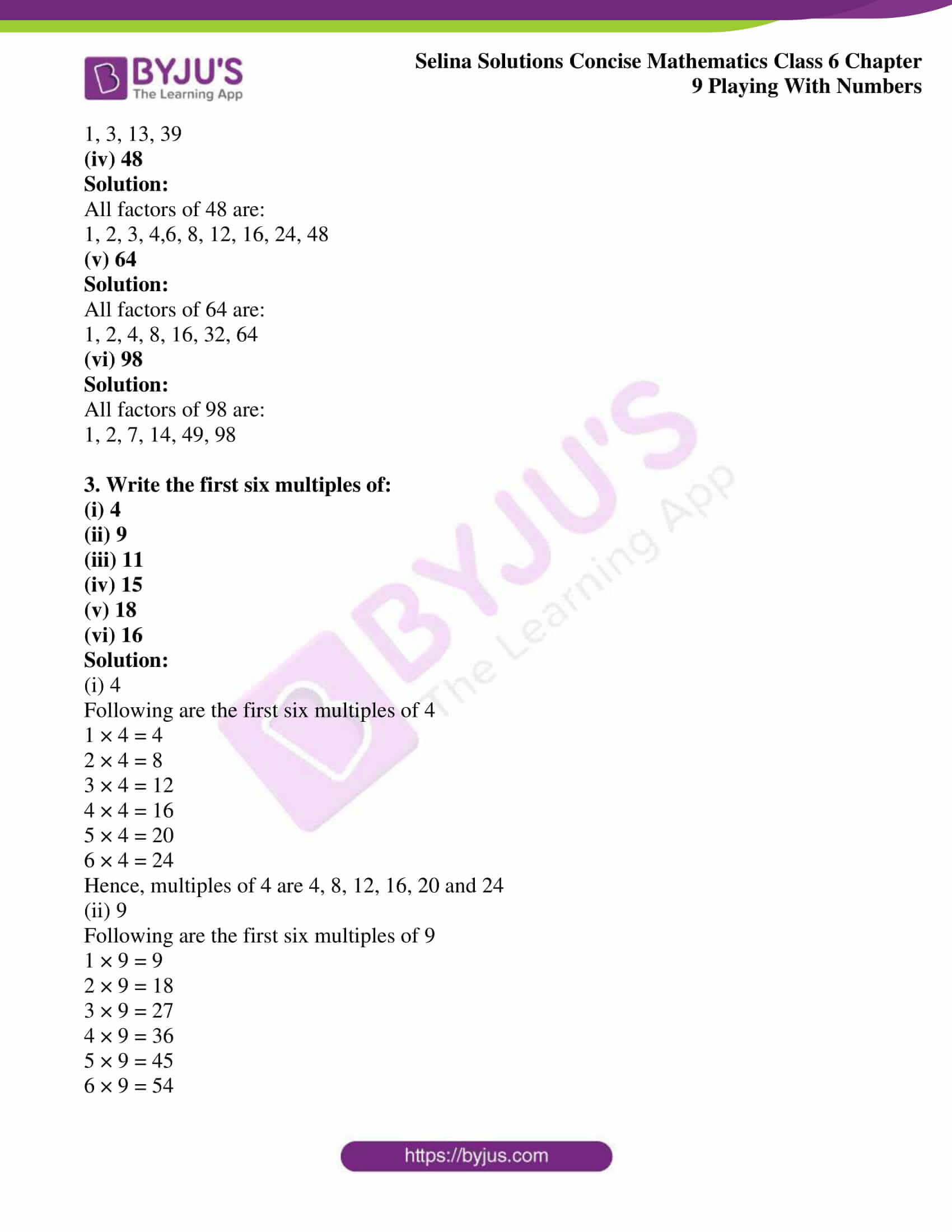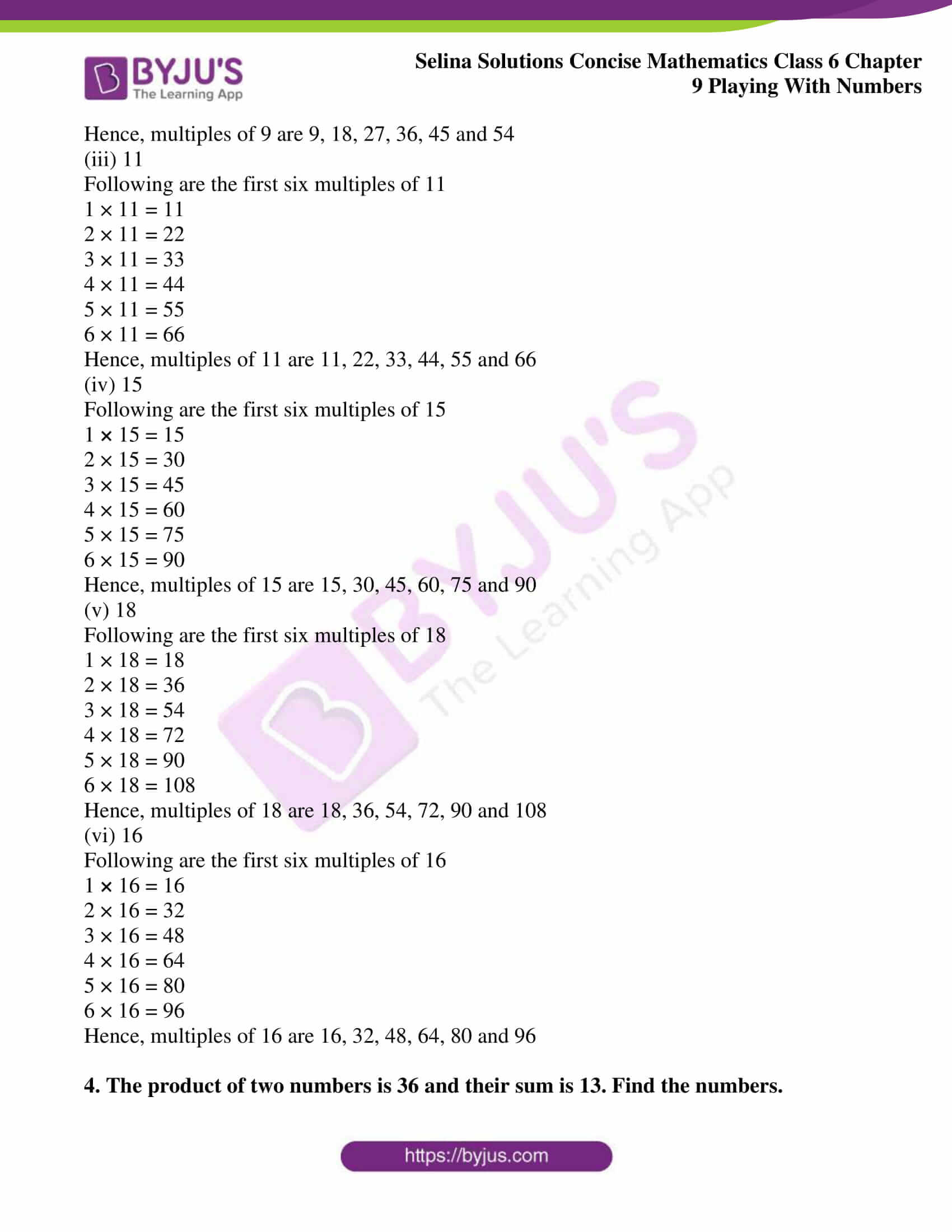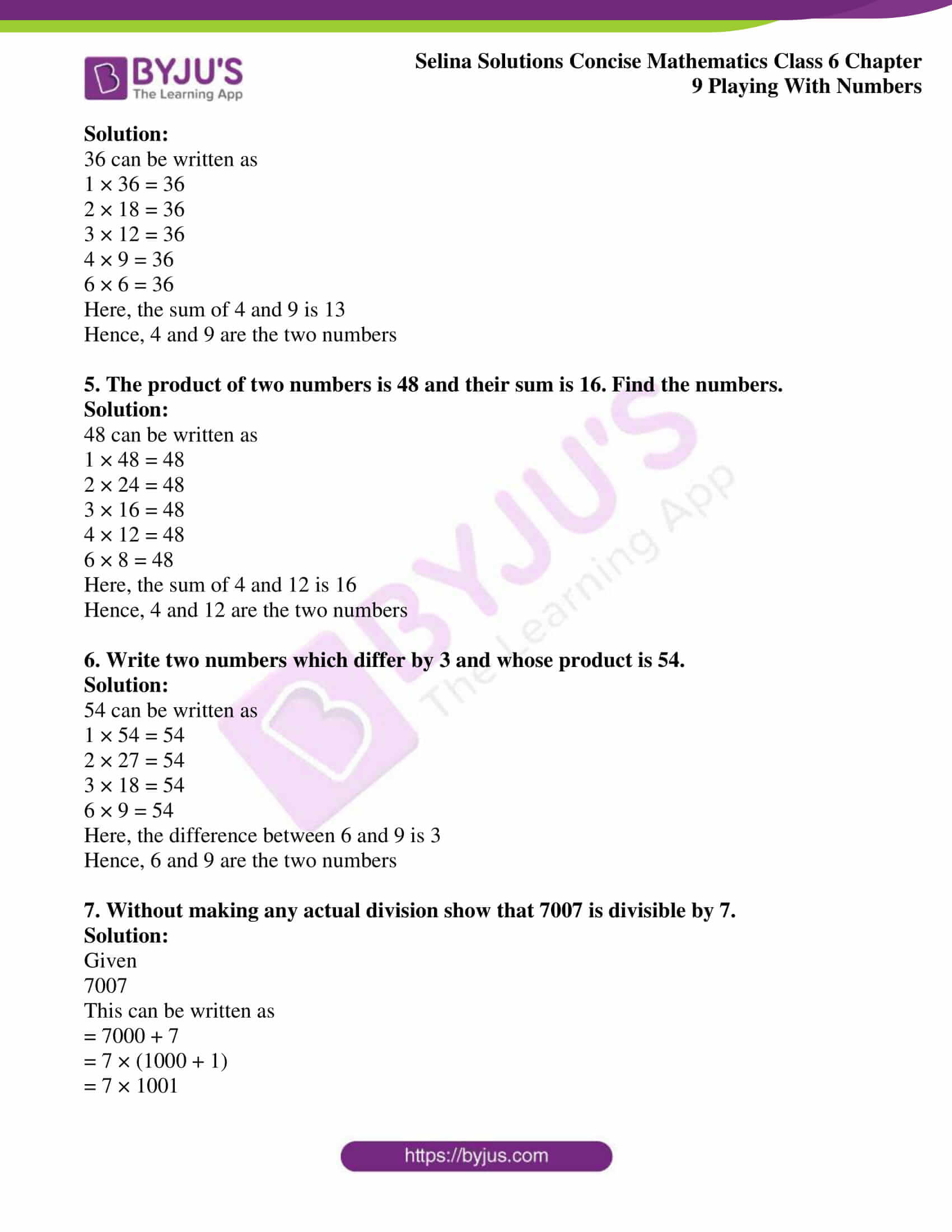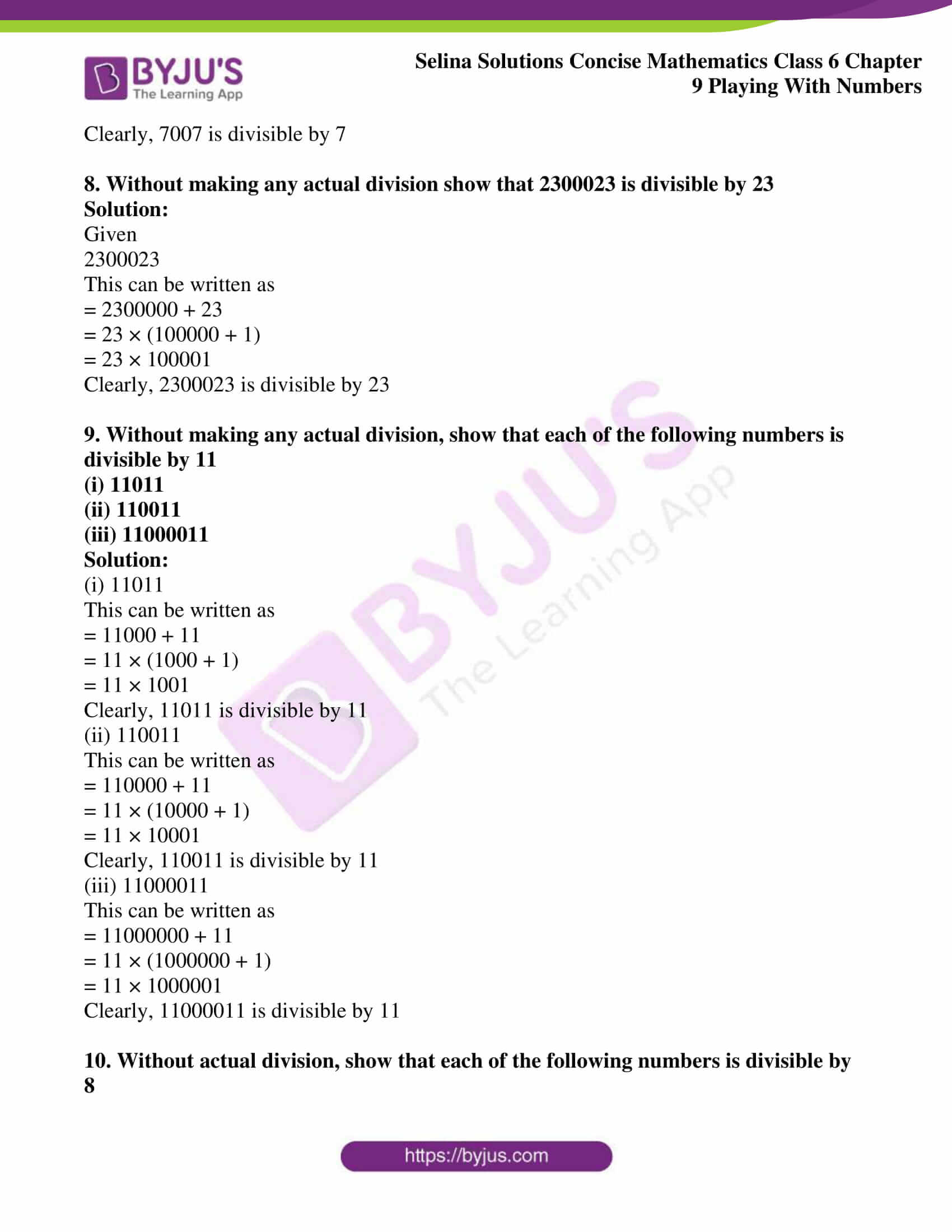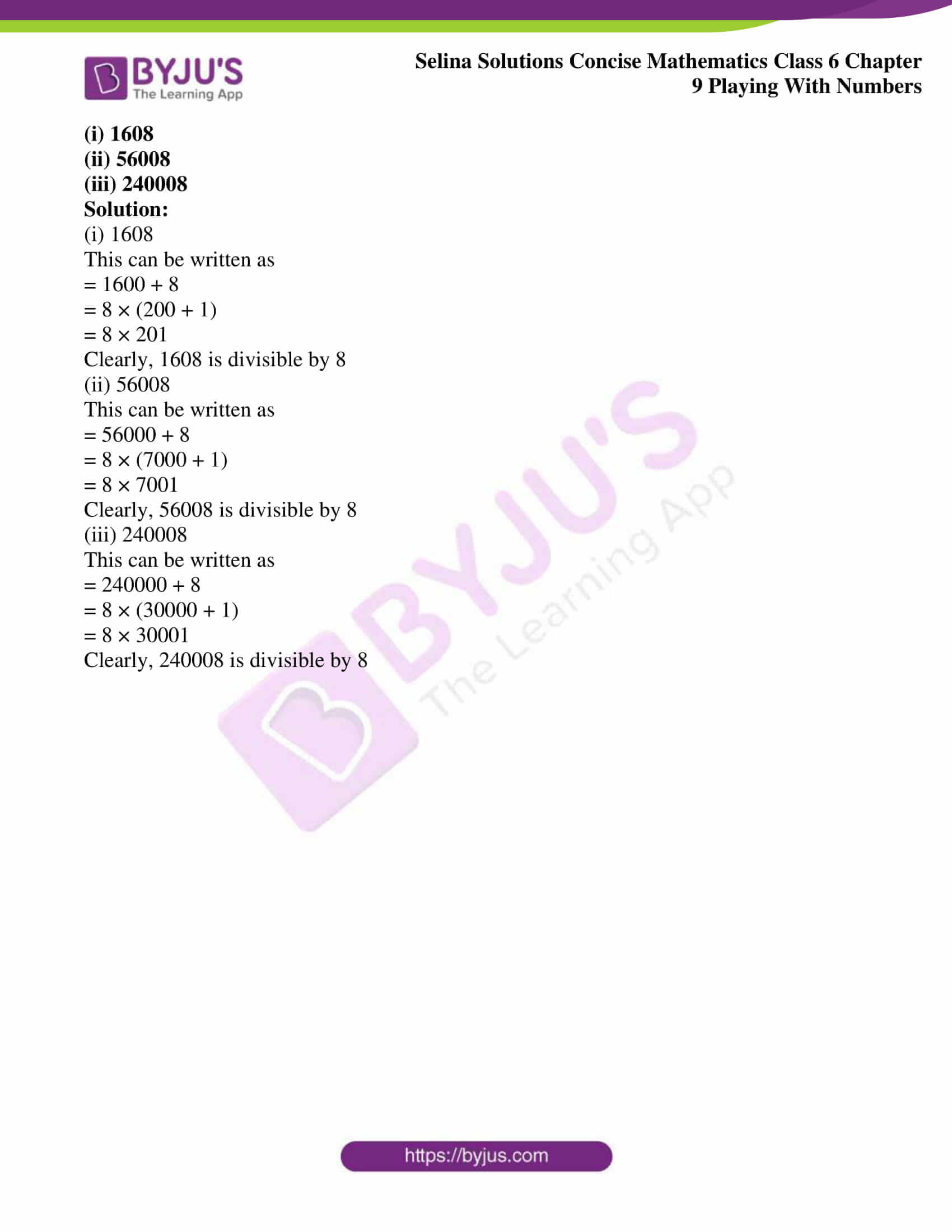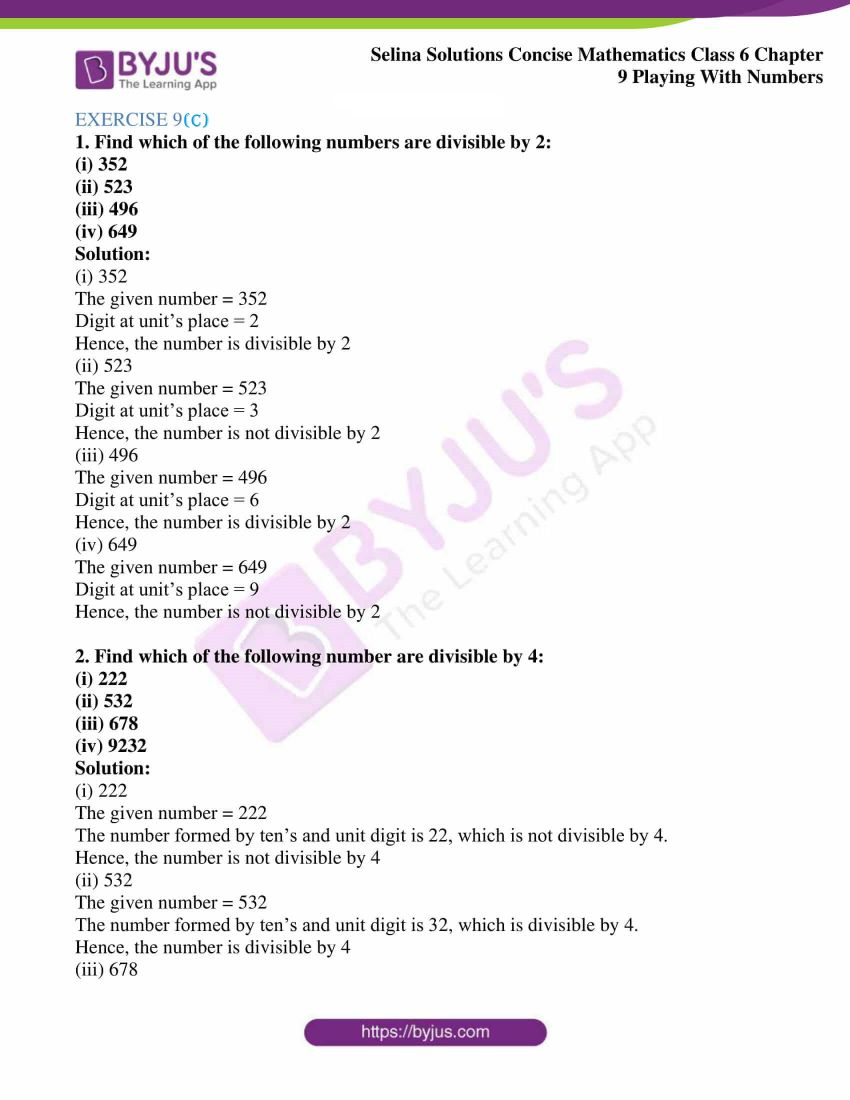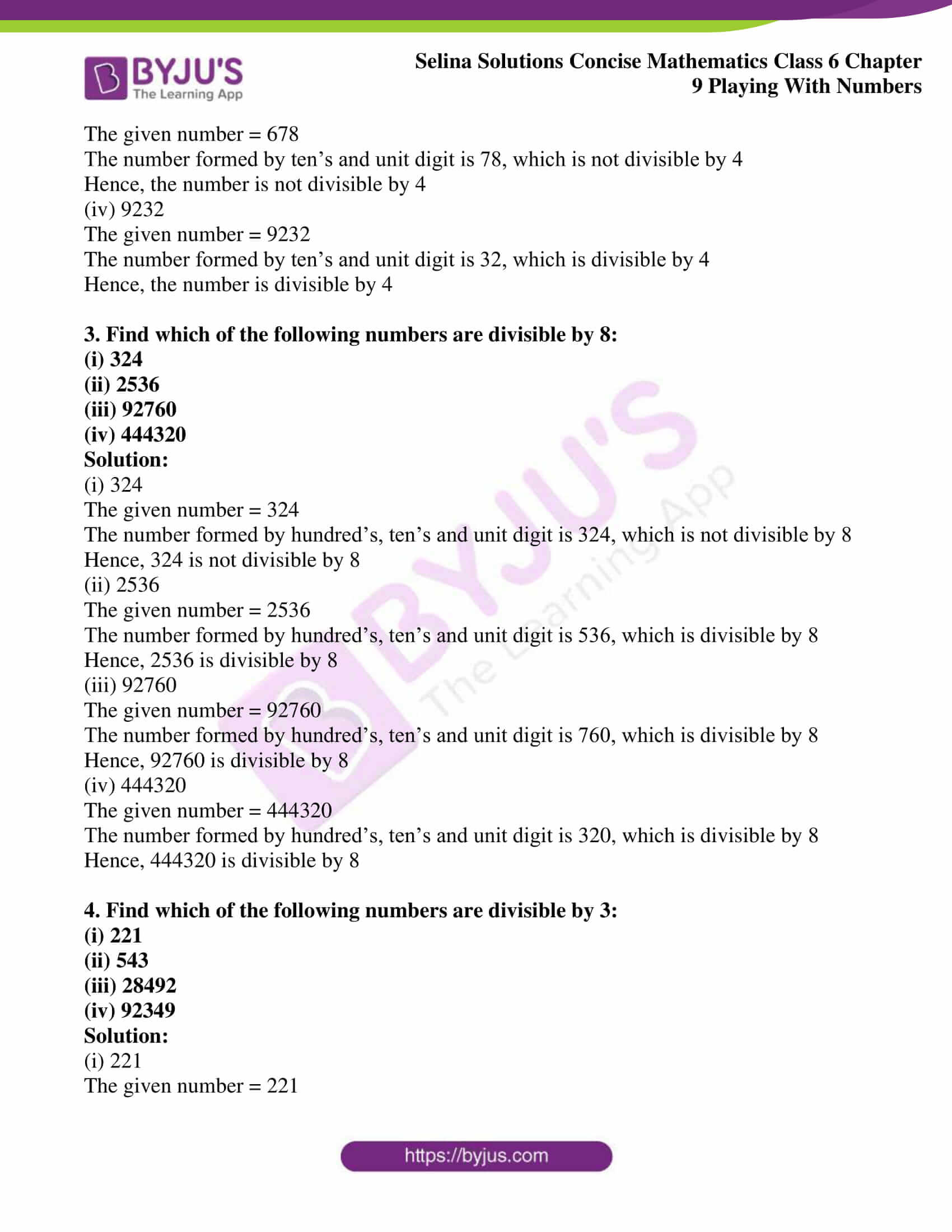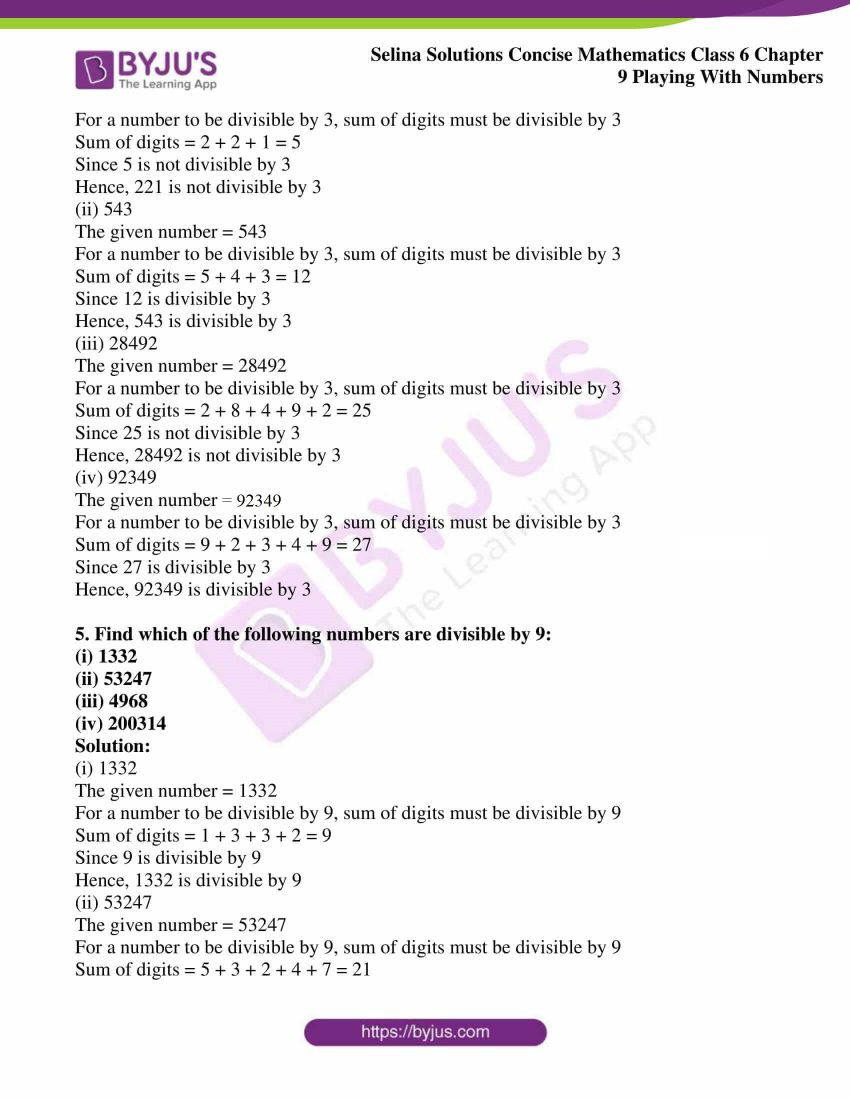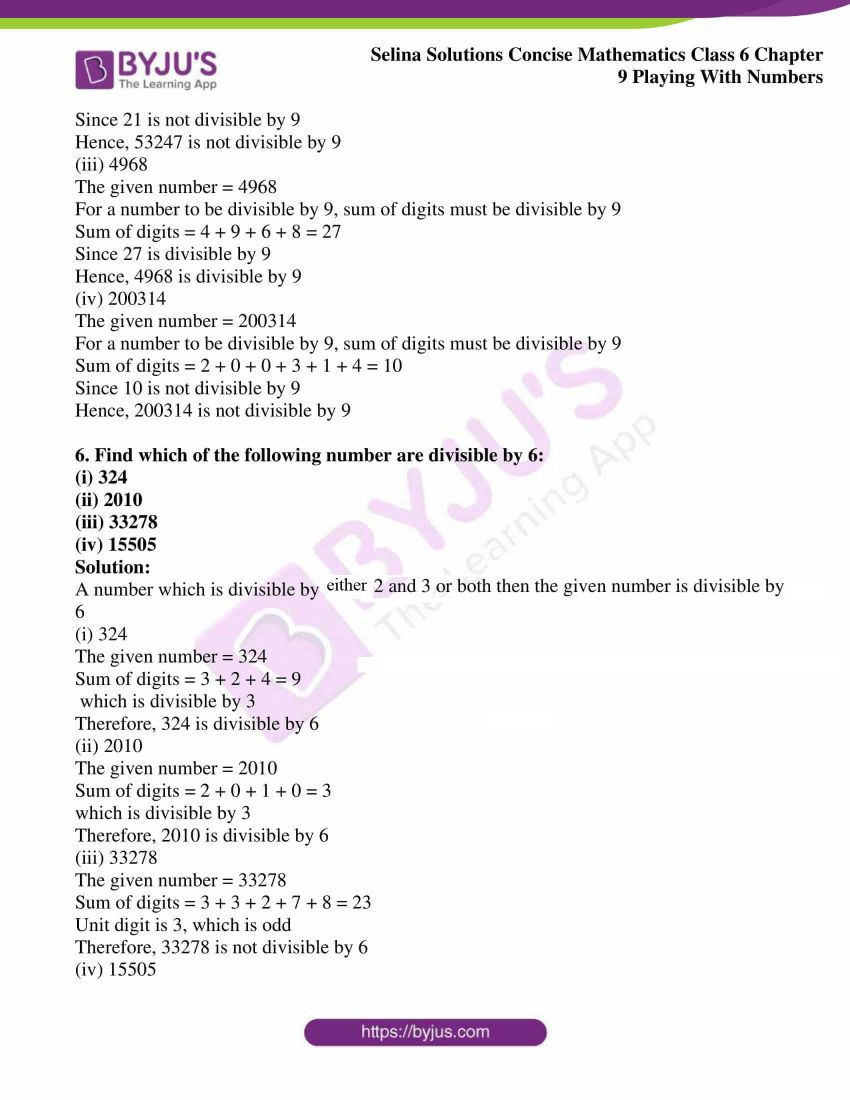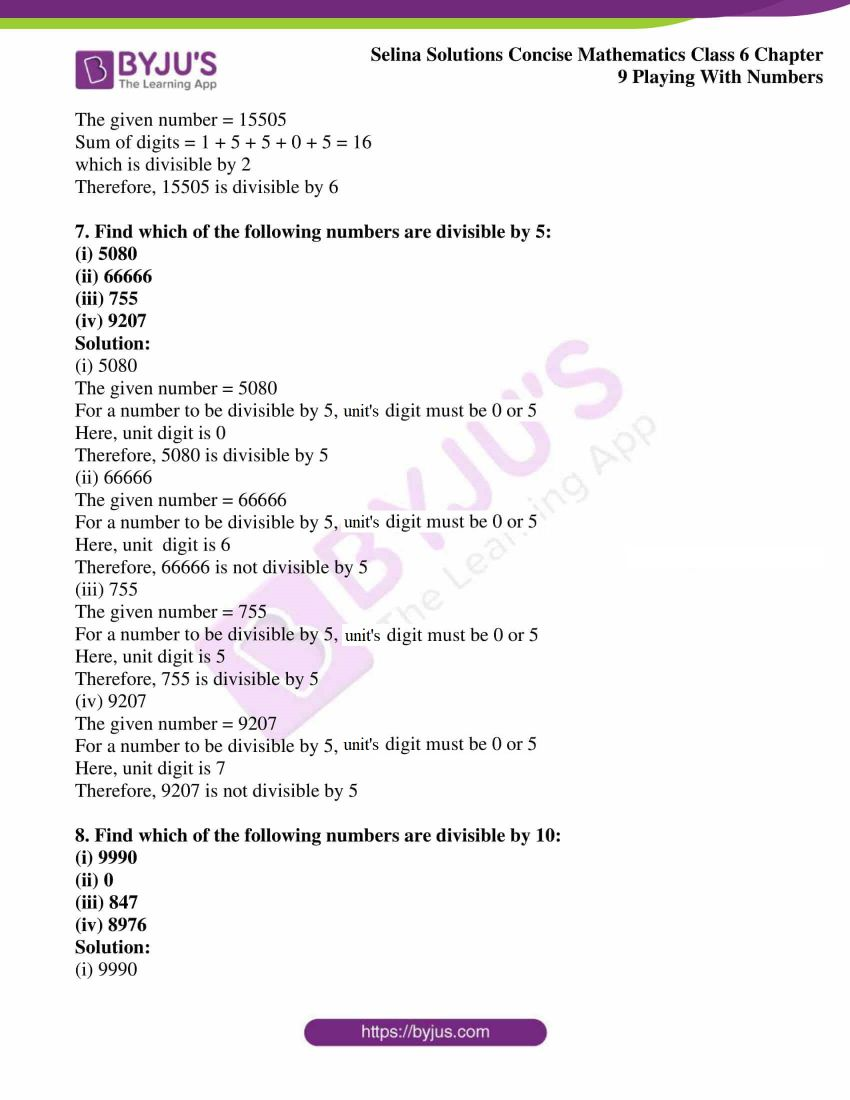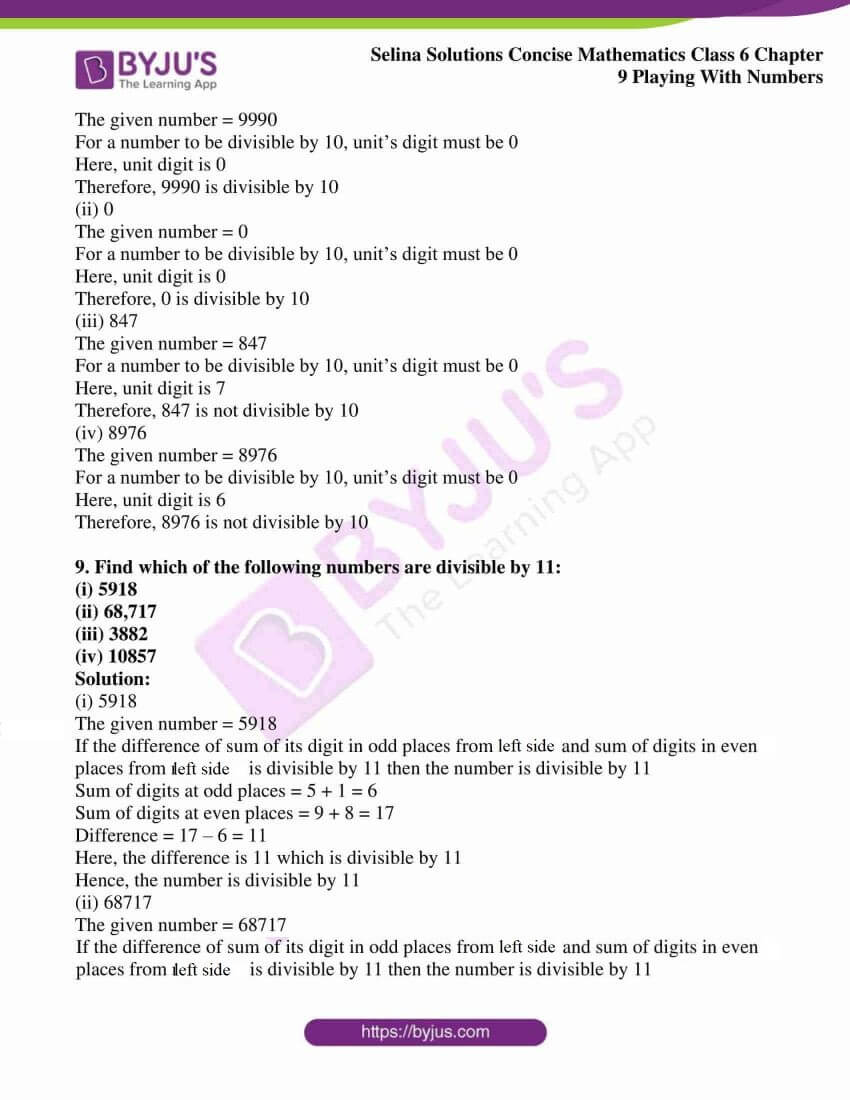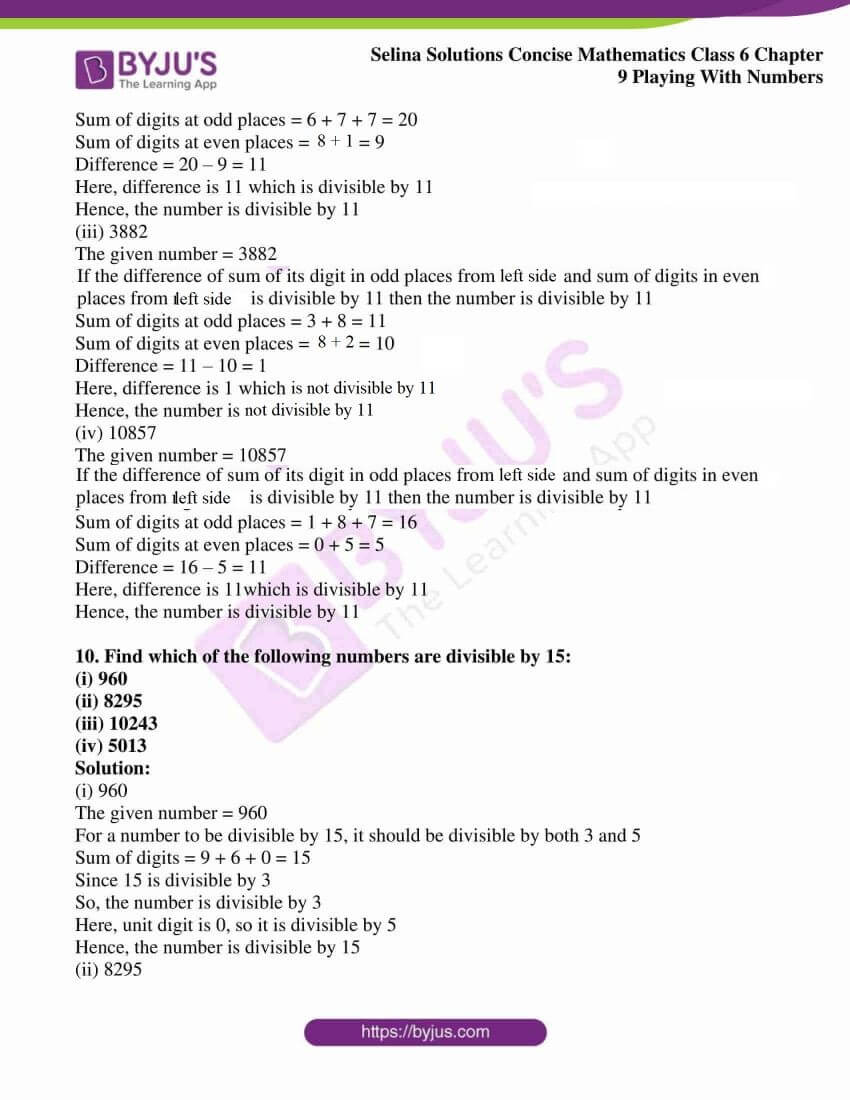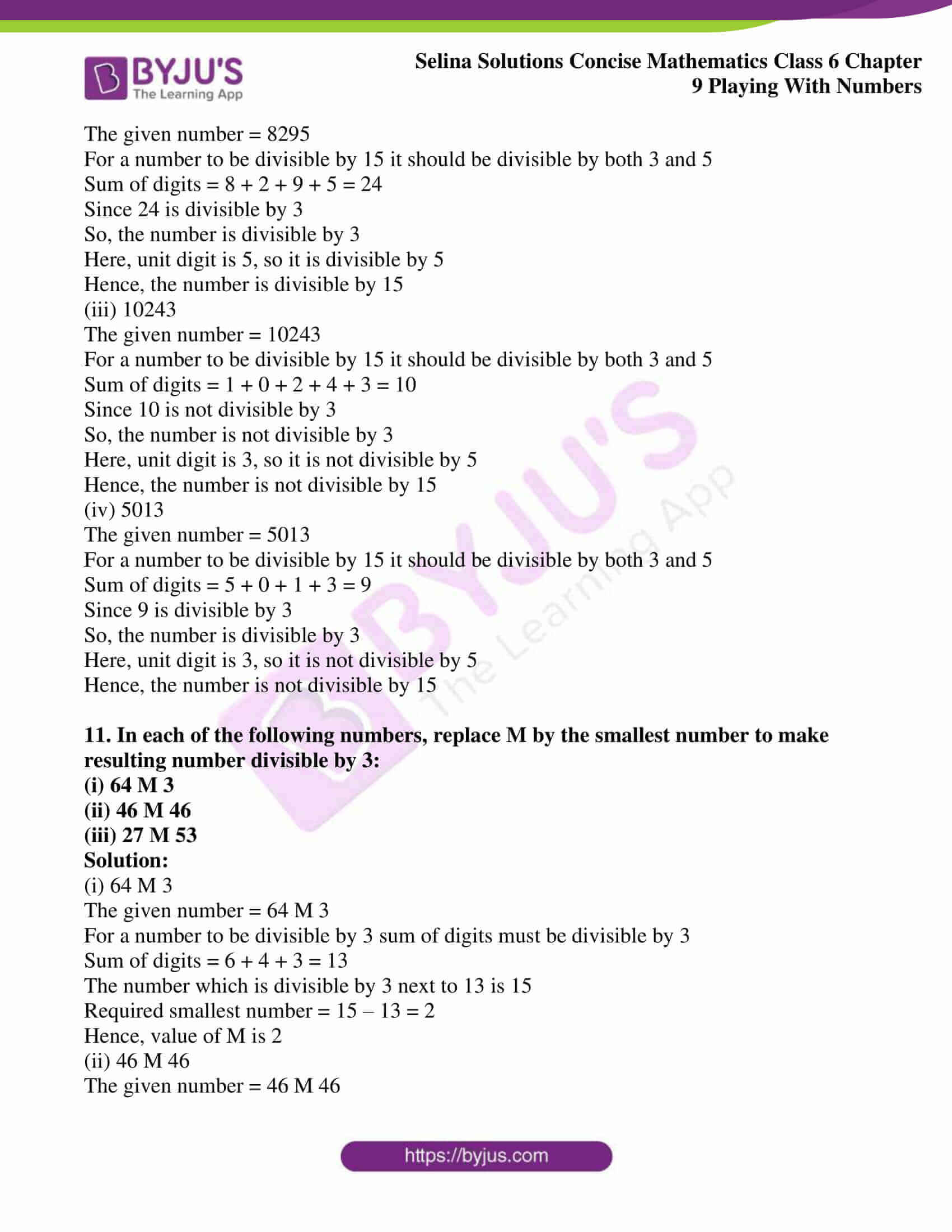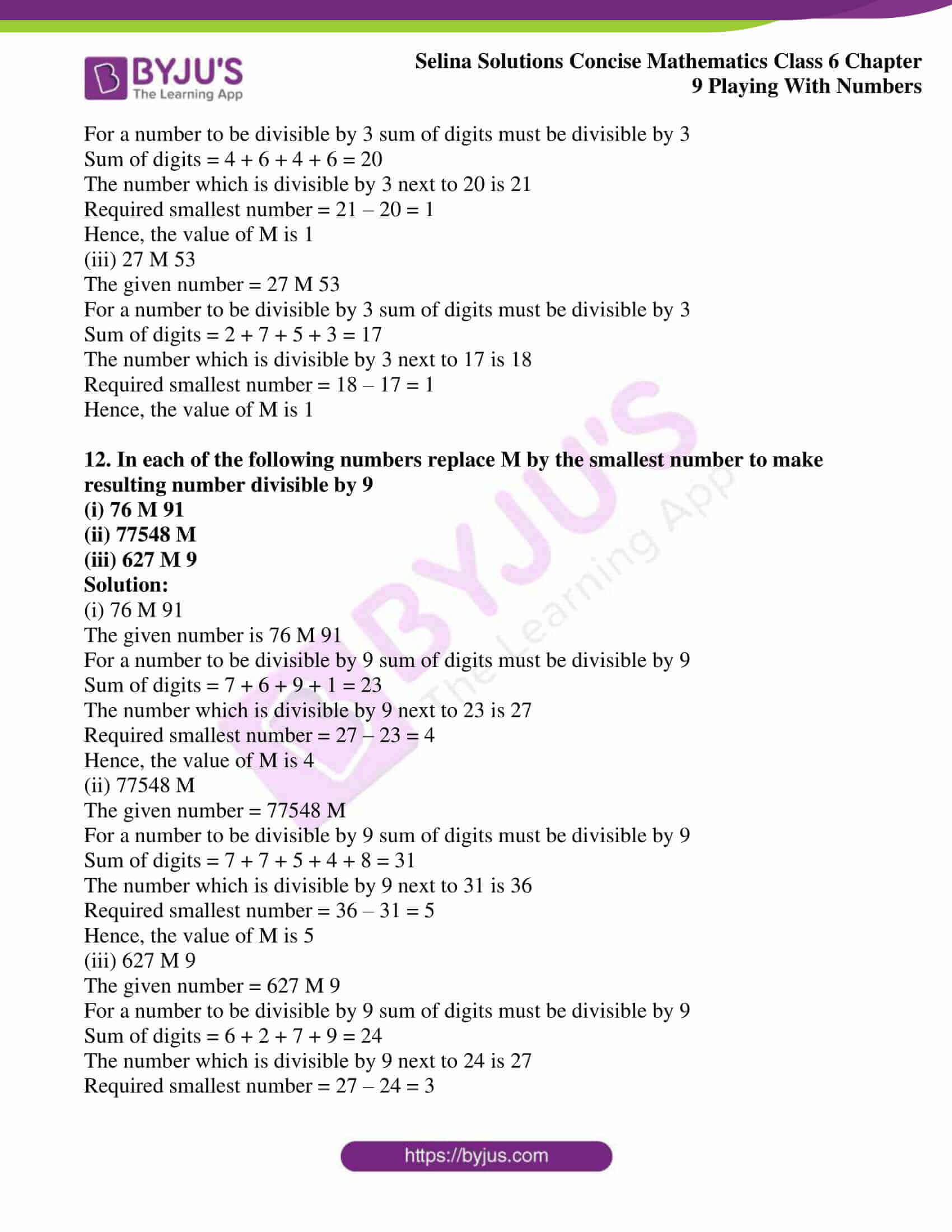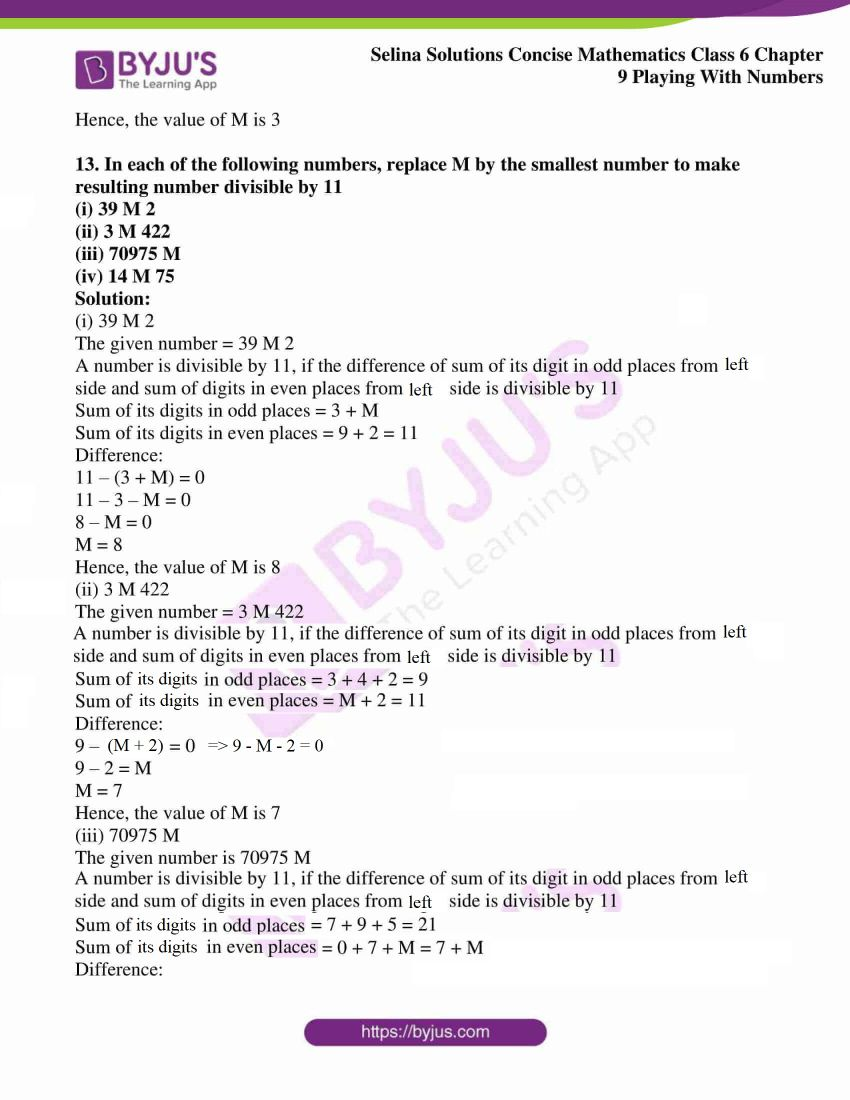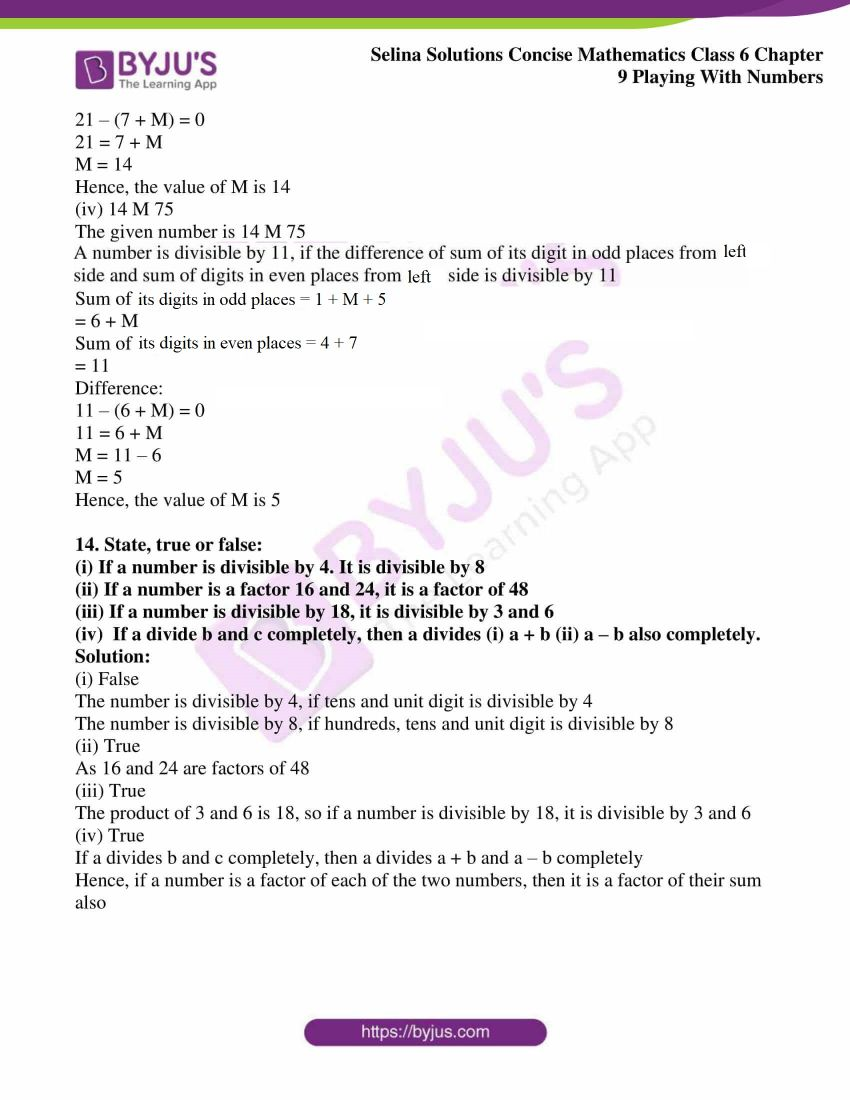### Exercises of Selina Solutions Concise Mathematics Class 6 Chapter 9: Playing With Numbers

Exercise 9(A) Solutions

Exercise 9(B) Solutions

Exercise 9(C) Solutions

## Access Selina Solutions Concise Mathematics Class 6 Chapter 9: Playing With Numbers

#### Exercise 9(A)

(Using BODMOS)

1. 19 – (1 + 5) – 3

Solution:

Given

19 – (1 + 5) – 3

On further calculation, we get

= 19 – 6 – 3

= 19 – 9

= 10

2. 30 × 6 ÷ (5 – 2)

Solution:

Given

30 × 6 ÷ (5 – 2)

On further calculation, we get

= 30 × 6 ÷ 3

= 30 × 2

= 60

3. 28 – (3 × 8) ÷ 6

Solution:

Given

28 – (3 × 8) ÷ 6

Calculating further, we get

= 28 – 24 ÷ 6

= 28 – 4

= 24

4. 9 – [(4 – 3) + 2 × 5]

Solution:

Given

9 – [(4 – 3) + 2 × 5]

We get

= 9 – [1 + 10]

= 9 – 11

= – 2

5. [18 – (15 ÷ 5) + 6]

Solution:

Given

[18 – (15 ÷ 5) + 6]

On further calculation, we get

= [18 – 3 + 6]

= 18 + 3

= 21

6. [(4 × 2) – (4 ÷ 2)] + 8

Solution:

Given

[(4 × 2) – (4 ÷ 2)] + 8

On further calculation, we get

= [8 – 2] + 8

= 6 + 8

= 14

7. 48 + 96 ÷ 24 – 6 × 18

Solution:

Given

48 + 96 ÷ 24 – 6 × 18

We get

= 48 + 4 – 6 × 18

= 48 + 4 – 108

= 52 – 108

= – 56

8. 22 – [3 – {8 – (4 + 6)}]

Solution:

Given

22 – [3 – {8 – (4 + 6)}]

On calculating further, we get

= 22 – [3 – {8 – 10}]

= 22 – [3 + 2]

= 22 – 5

= 17

9. 34 – [29 – {30 + 66 ÷ (24 –

$$\begin{array}{l}\overline{28 – 26}\end{array}$$
)}]

Solution:

Given

34 – [29 – {30 + 66 ÷ (24 –

$$\begin{array}{l}\overline{28 – 26}\end{array}$$
)}]

On further calculation, we get

= 34 – [29 – {30 + 66 ÷ (24 – 2)}]

= 34 – [29 – {30 + 66 ÷ 22}]

= 34 – [29 – {30 + 3}]

= 34 – [29 – 33]

= 34 – [-4]

= 34 + 4

= 38

10. 60 – {16 ÷ (4 × 6 – 8)}

Solution:

Given

60 – {16 ÷ (4 × 6 – 8)}

On further calculation, we get

= 60 – {16 ÷ (24 – 8)}

= 60 – {16 ÷ 16}

= 60 – 1

= 59

11. 25 – [12 – {5 + 18 ÷ (4 –

$$\begin{array}{l}\overline{5 – 3}\end{array}$$
)}]

Solution:

Given

25 – [12 – {5 + 18 ÷ (4 –

$$\begin{array}{l}\overline{5 – 3}\end{array}$$
)}]

By calculating further, we get

= 25 – [12 – {5 + 18 ÷ (4 – 2)}]

= 25 – [12 – {5 + 18 ÷ 2}]

= 25 – [12 – {5 + 9}]

= 25 – [12 – 14]

= 25 – [-2]

= 25 + 2

= 27

12. 15 – [16 – {12 + 21 ÷ (9 – 2)}]

Solution:

Given

15 – [16 – {12 + 21 ÷ (9 – 2)}]

On further calculation, we get

= 15 – [16 – {12 + 21 ÷ 7}]

= 15 – [16 – {12 + 3}]

= 15 – [16 – 15]

= 15 – 1

= 14

#### Exercise 9(B)

1. Fill in the blanks:

(i) On dividing 9 by 7, quotient = …………….. and remainder = …………….

(ii) On dividing 18 by 6, quotient = ………….. and remainder = …………

(iii) Factor of a number is ………….. of ………..

(iv) Every number is a factor of ………….

(v) Every number is a multiple of …………

(vi) ………. is factor of every number.

(vii) For every number, its factors are ………. and its multiples are ……….

(viii) x is a factor of y, then y is a …….. of x.

Solution:

(i) On dividing 9 by 7, quotient = 1 and remainder = 2

(ii) On dividing 18 by 6, quotient = 3 and remainder = 0

(iii) Factor of a number is an exact division of the number

(iv) Every number is a factor of itself

(v) Every number is a multiple of itself

(vi) One is factor of every number

(vii) For every number, its factors are finite and its multiples are infinite

(viii) x is a factor of y, then y is a multiple of x

2. Write all the factors of:

(i) 16

(ii) 21

(iii) 39

(iv) 48

(v) 64

(vi) 98

Solution:

(i) 16

Solution:

All factors of 16 are:

1, 2, 4, 8, 16

(ii) 21

Solution:

All factors of 21 are:

1, 3, 7, 21

(iii) 39

Solution:

All factors of 39 are:

1, 3, 13, 39

(iv) 48

Solution:

All factors of 48 are:

1, 2, 3, 4,6, 8, 12, 16, 24, 48

(v) 64

Solution:

All factors of 64 are:

1, 2, 4, 8, 16, 32, 64

(vi) 98

Solution:

All factors of 98 are:

1, 2, 7, 14, 49, 98

3. Write the first six multiples of:

(i) 4

(ii) 9

(iii) 11

(iv) 15

(v) 18

(vi) 16

Solution:

(i) 4

Following are the first six multiples of 4

1 × 4 = 4

2 × 4 = 8

3 × 4 = 12

4 × 4 = 16

5 × 4 = 20

6 × 4 = 24

Hence, multiples of 4 are 4, 8, 12, 16, 20 and 24

(ii) 9

Following are the first six multiples of 9

1 × 9 = 9

2 × 9 = 18

3 × 9 = 27

4 × 9 = 36

5 × 9 = 45

6 × 9 = 54

Hence, multiples of 9 are 9, 18, 27, 36, 45 and 54

(iii) 11

Following are the first six multiples of 11

1 × 11 = 11

2 × 11 = 22

3 × 11 = 33

4 × 11 = 44

5 × 11 = 55

6 × 11 = 66

Hence, multiples of 11 are 11, 22, 33, 44, 55 and 66

(iv) 15

Following are the first six multiples of 15

1 × 15 = 15

2 × 15 = 30

3 × 15 = 45

4 × 15 = 60

5 × 15 = 75

6 × 15 = 90

Hence, multiples of 15 are 15, 30, 45, 60, 75 and 90

(v) 18

Following are the first six multiples of 18

1 × 18 = 18

2 × 18 = 36

3 × 18 = 54

4 × 18 = 72

5 × 18 = 90

6 × 18 = 108

Hence, multiples of 18 are 18, 36, 54, 72, 90 and 108

(vi) 16

Following are the first six multiples of 16

1 × 16 = 16

2 × 16 = 32

3 × 16 = 48

4 × 16 = 64

5 × 16 = 80

6 × 16 = 96

Hence, multiples of 16 are 16, 32, 48, 64, 80 and 96

4. The product of two numbers is 36 and their sum is 13. Find the numbers.

Solution:

36 can be written as

1 × 36 = 36

2 × 18 = 36

3 × 12 = 36

4 × 9 = 36

6 × 6 = 36

Here, the sum of 4 and 9 is 13

Hence, 4 and 9 are the two numbers

5. The product of two numbers is 48 and their sum is 16. Find the numbers.

Solution:

48 can be written as

1 × 48 = 48

2 × 24 = 48

3 × 16 = 48

4 × 12 = 48

6 × 8 = 48

Here, the sum of 4 and 12 is 16

Hence, 4 and 12 are the two numbers

6. Write two numbers which differ by 3 and whose product is 54.

Solution:

54 can be written as

1 × 54 = 54

2 × 27 = 54

3 × 18 = 54

6 × 9 = 54

Here, the difference between 6 and 9 is 3

Hence, 6 and 9 are the two numbers

7. Without making any actual division show that 7007 is divisible by 7.

Solution:

Given

7007

This can be written as

= 7000 + 7

= 7 × (1000 + 1)

= 7 × 1001

Clearly, 7007 is divisible by 7

8. Without making any actual division show that 2300023 is divisible by 23

Solution:

Given

2300023

This can be written as

= 2300000 + 23

= 23 × (100000 + 1)

= 23 × 100001

Clearly, 2300023 is divisible by 23

9. Without making any actual division, show that each of the following numbers is divisible by 11

(i) 11011

(ii) 110011

(iii) 11000011

Solution:

(i) 11011

This can be written as

= 11000 + 11

= 11 × (1000 + 1)

= 11 × 1001

Clearly, 11011 is divisible by 11

(ii) 110011

This can be written as

= 110000 + 11

= 11 × (10000 + 1)

= 11 × 10001

Clearly, 110011 is divisible by 11

(iii) 11000011

This can be written as

= 11000000 + 11

= 11 × (1000000 + 1)

= 11 × 1000001

Clearly, 11000011 is divisible by 11

10. Without actual division, show that each of the following numbers is divisible by 8

(i) 1608

(ii) 56008

(iii) 240008

Solution:

(i) 1608

This can be written as

= 1600 + 8

= 8 × (200 + 1)

= 8 × 201

Clearly, 1608 is divisible by 8

(ii) 56008

This can be written as

= 56000 + 8

= 8 × (7000 + 1)

= 8 × 7001

Clearly, 56008 is divisible by 8

(iii) 240008

This can be written as

= 240000 + 8

= 8 × (30000 + 1)

= 8 × 30001

Clearly, 240008 is divisible by 8

#### Exercise 9(C)

1. Find which of the following numbers are divisible by 2:

(i) 352

(ii) 523

(iii) 496

(iv) 649

Solution:

(i) 352

The given number = 352

Digit at unit’s place = 2

Hence, the number is divisible by 2

(ii) 523

The given number = 523

Digit at unit’s place = 3

Hence, the number is not divisible by 2

(iii) 496

The given number = 496

Digit at unit’s place = 6

Hence, the number is divisible by 2

(iv) 649

The given number = 649

Digit at unit’s place = 9

Hence, the number is not divisible by 2

2. Find which of the following number are divisible by 4:

(i) 222

(ii) 532

(iii) 678

(iv) 9232

Solution:

(i) 222

The given number = 222

The number formed by ten’s and unit digit is 22, which is not divisible by 4.

Hence, the number is not divisible by 4

(ii) 532

The given number = 532

The number formed by ten’s and unit digit is 32, which is divisible by 4.

Hence, the number is divisible by 4

(iii) 678

The given number = 678

The number formed by ten’s and unit digit is 78, which is not divisible by 4

Hence, the number is not divisible by 4

(iv) 9232

The given number = 9232

The number formed by ten’s and unit digit is 32, which is divisible by 4

Hence, the number is divisible by 4

3. Find which of the following numbers are divisible by 8:

(i) 324

(ii) 2536

(iii) 92760

(iv) 444320

Solution:

(i) 324

The given number = 324

The number formed by hundred’s, ten’s and unit digit is 324, which is not divisible by 8

Hence, 324 is not divisible by 8

(ii) 2536

The given number = 2536

The number formed by hundred’s, ten’s and unit digit is 536, which is divisible by 8

Hence, 2536 is divisible by 8

(iii) 92760

The given number = 92760

The number formed by hundred’s, ten’s and unit digit is 760, which is divisible by 8

Hence, 92760 is divisible by 8

(iv) 444320

The given number = 444320

The number formed by hundred’s, ten’s and unit digit is 320, which is divisible by 8

Hence, 444320 is divisible by 8

4. Find which of the following numbers are divisible by 3:

(i) 221

(ii) 543

(iii) 28492

(iv) 92349

Solution:

(i) 221

The given number = 221

For a number to be divisible by 3, sum of digits must be divisible by 3

Sum of digits = 2 + 2 + 1 = 5

Since 5 is not divisible by 3

Hence, 221 is not divisible by 3

(ii) 543

The given number = 543

For a number to be divisible by 3, sum of digits must be divisible by 3

Sum of digits = 5 + 4 + 3 = 12

Since 12 is divisible by 3

Hence, 543 is divisible by 3

(iii) 28492

The given number = 28492

For a number to be divisible by 3, sum of digits must be divisible by 3

Sum of digits = 2 + 8 + 4 + 9 + 2 = 25

Since 25 is not divisible by 3

Hence, 28492 is not divisible by 3

(iv) 92349

The given number = 92349

For a number to be divisible by 3, sum of digits must be divisible by 3

Sum of digits = 9 + 2 + 3 + 4 + 9 = 27

Since 27 is divisible by 3

Hence, 92349 is divisible by 3

5. Find which of the following numbers are divisible by 9:

(i) 1332

(ii) 53247

(iii) 4968

(iv) 200314

Solution:

(i) 1332

The given number = 1332

For a number to be divisible by 9, sum of digits must be divisible by 9

Sum of digits = 1 + 3 + 3 + 2 = 9

Since 9 is divisible by 9

Hence, 1332 is divisible by 9

(ii) 53247

The given number = 53247

For a number to be divisible by 9, sum of digits must be divisible by 9

Sum of digits = 5 + 3 + 2 + 4 + 7 = 21

Since 21 is not divisible by 9

Hence, 53247 is not divisible by 9

(iii) 4968

The given number = 4968

For a number to be divisible by 9, sum of digits must be divisible by 9

Sum of digits = 4 + 9 + 6 + 8 = 27

Since 27 is divisible by 9

Hence, 4968 is divisible by 9

(iv) 200314

The given number = 200314

For a number to be divisible by 9, sum of digits must be divisible by 9

Sum of digits = 2 + 0 + 0 + 3 + 1 + 4 = 10

Since 10 is not divisible by 9

Hence, 200314 is not divisible by 9

6. Find which of the following number are divisible by 6:

(i) 324

(ii) 2010

(iii) 33278

(iv) 15505

Solution:

A number which is divisible by either 2 and 3 or both then the given number is divisible by 6

(i) 324

The given number = 324

Sum of digits = 3 + 2 + 4 = 9

which is divisible by 3

Therefore, 324 is divisible by 6

(ii) 2010

The given number = 2010

Sum of digits = 2 + 0 + 1 + 0 = 3

which is divisible by 3

Therefore, 2010 is divisible by 6

(iii) 33278

The given number = 33278

Sum of digits = 3 + 3 + 2 + 7 + 8 = 23

Unit digit is 3, which is odd

Therefore, 33278 is not divisible by 6

(iv) 15505

The given number = 15505

Sum of digits = 1 + 5 + 5 + 0 + 5 = 16

which is divisible by 2

Therefore, 15505 is divisible by 6

7. Find which of the following numbers are divisible by 5:

(i) 5080

(ii) 66666

(iii) 755

(iv) 9207

Solution:

(i) 5080

The given number = 5080

For a number to be divisible by 5, unit’s digit must be 0 or 5

Here, unit digit is 0

Therefore, 5080 is divisible by 5

(ii) 66666

The given number = 66666

For a number to be divisible by 5, unit’s digit must be 0 or 5

Here, unit digit is 6

Therefore, 66666 is not divisible by 5

(iii) 755

The given number = 755

For a number to be divisible by 5, unit’s digit must be 0 or 5

Here, unit digit is 5

Therefore, 755 is divisible by 5

(iv) 9207

The given number = 9207

For a number to be divisible by 5, unit’s digit must be 0 or 5

Here, unit digit is 7

Therefore, 9207 is not divisible by 5

8. Find which of the following numbers are divisible by 10:

(i) 9990

(ii) 0

(iii) 847

(iv) 8976

Solution:

(i) 9990

The given number = 9990

For a number to be divisible by 10, unit’s digit must be 0

Here, unit digit is 0

Therefore, 9990 is divisible by 10

(ii) 0

The given number = 0

For a number to be divisible by 10, unit’s digit must be 0

Here, unit digit is 0

Therefore, 0 is divisible by 10

(iii) 847

The given number = 847

For a number to be divisible by 10, unit’s digit must be 0

Here, unit digit is 7

Therefore, 847 is not divisible by 10

(iv) 8976

The given number = 8976

For a number to be divisible by 10, unit’s digit must be 0

Here, unit digit is 6

Therefore, 8976 is not divisible by 10

9. Find which of the following numbers are divisible by 11:

(i) 5918

(ii) 68,717

(iii) 3882

(iv) 10857

Solution:

(i) 5918

The given number = 5918

If the difference of sum of its digit in odd places from left side and sum of digits in even places from left side is divisible by 11 then the number is divisible by 11

Sum of digits at odd places = 5 + 1 = 6

Sum of digits at even places = 9 + 8 = 17

Difference = 17 – 6 = 11

Here, the difference is 11 which is divisible by 11

Hence, the number is divisible by 11

(ii) 68717

The given number = 68717

If the difference of sum of its digit in odd places from left side and sum of digits in even places from left side is divisible by 11 then the number is divisible by 11

Sum of digits at odd places = 6 + 7 + 7 = 20

Sum of digits at even places = 8 + 1 = 9

Difference = 20 – 9 = 11

Here, difference is 11 which is divisible by 11

Hence, the number is divisible by 11

(iii) 3882

The given number = 3882

If the difference of sum of its digit in odd places from left side and sum of digits in even places from left side is divisible by 11 then the number is divisible by 11

Sum of digits at odd places = 3 + 8 = 11

Sum of digits at even places = 8 + 2 = 10

Difference = 11 – 10 = 1

Here, difference is 1 which is not divisible by 11

Hence, the number is not divisible by 11

(iv) 10857

The given number = 10857

If the difference of sum of its digit in odd places from left side and sum of digits in even places from left side is divisible by 11 then the number is divisible by 11

Sum of digits at odd places = 1 + 8 + 7 = 16

Sum of digits at even places = 0 + 5 = 5

Difference = 16 – 5 = 11

Here, difference is 11which is divisible by 11

Hence, the number is divisible by 11

10. Find which of the following numbers are divisible by 15:

(i) 960

(ii) 8295

(iii) 10243

(iv) 5013

Solution:

(i) 960

The given number = 960

For a number to be divisible by 15, it should be divisible by both 3 and 5

Sum of digits = 9 + 6 + 0 = 15

Since 15 is divisible by 3

So, the number is divisible by 3

Here, unit digit is 0, so it is divisible by 5

Hence, the number is divisible by 15

(ii) 8295

The given number = 8295

For a number to be divisible by 15 it should be divisible by both 3 and 5

Sum of digits = 8 + 2 + 9 + 5 = 24

Since 24 is divisible by 3

So, the number is divisible by 3

Here, unit digit is 5, so it is divisible by 5

Hence, the number is divisible by 15

(iii) 10243

The given number = 10243

For a number to be divisible by 15 it should be divisible by both 3 and 5

Sum of digits = 1 + 0 + 2 + 4 + 3 = 10

Since 10 is not divisible by 3

So, the number is not divisible by 3

Here, unit digit is 3, so it is not divisible by 5

Hence, the number is not divisible by 15

(iv) 5013

The given number = 5013

For a number to be divisible by 15 it should be divisible by both 3 and 5

Sum of digits = 5 + 0 + 1 + 3 = 9

Since 9 is divisible by 3

So, the number is divisible by 3

Here, unit digit is 3, so it is not divisible by 5

Hence, the number is not divisible by 15

11. In each of the following numbers, replace M by the smallest number to make resulting number divisible by 3:

(i) 64 M 3

(ii) 46 M 46

(iii) 27 M 53

Solution:

(i) 64 M 3

The given number = 64 M 3

For a number to be divisible by 3 sum of digits must be divisible by 3

Sum of digits = 6 + 4 + 3 = 13

The number which is divisible by 3 next to 13 is 15

Required smallest number = 15 – 13 = 2

Hence, value of M is 2

(ii) 46 M 46

The given number = 46 M 46

For a number to be divisible by 3 sum of digits must be divisible by 3

Sum of digits = 4 + 6 + 4 + 6 = 20

The number which is divisible by 3 next to 20 is 21

Required smallest number = 21 – 20 = 1

Hence, the value of M is 1

(iii) 27 M 53

The given number = 27 M 53

For a number to be divisible by 3 sum of digits must be divisible by 3

Sum of digits = 2 + 7 + 5 + 3 = 17

The number which is divisible by 3 next to 17 is 18

Required smallest number = 18 – 17 = 1

Hence, the value of M is 1

12. In each of the following numbers replace M by the smallest number to make resulting number divisible by 9

(i) 76 M 91

(ii) 77548 M

(iii) 627 M 9

Solution:

(i) 76 M 91

The given number is 76 M 91

For a number to be divisible by 9 sum of digits must be divisible by 9

Sum of digits = 7 + 6 + 9 + 1 = 23

The number which is divisible by 9 next to 23 is 27

Required smallest number = 27 – 23 = 4

Hence, the value of M is 4

(ii) 77548 M

The given number = 77548 M

For a number to be divisible by 9 sum of digits must be divisible by 9

Sum of digits = 7 + 7 + 5 + 4 + 8 = 31

The number which is divisible by 9 next to 31 is 36

Required smallest number = 36 – 31 = 5

Hence, the value of M is 5

(iii) 627 M 9

The given number = 627 M 9

For a number to be divisible by 9 sum of digits must be divisible by 9

Sum of digits = 6 + 2 + 7 + 9 = 24

The number which is divisible by 9 next to 24 is 27

Required smallest number = 27 – 24 = 3

Hence, the value of M is 3

13. In each of the following numbers, replace M by the smallest number to make resulting number divisible by 11

(i) 39 M 2

(ii) 3 M 422

(iii) 70975 M

(iv) 14 M 75

Solution:

(i) 39 M 2

The given number = 39 M 2

A number is divisible by 11, if the difference of sum of its digit in odd places from left side and sum of digits in even places from left side is divisible by 11

Sum of its digits in odd places = 3 + M

Sum of its digits in even places = 9 + 2 = 11

Difference:

11 – (3 + M) = 0

11 – 3 – M = 0

8 – M = 0

M = 8

Hence, the value of M is 8

(ii) 3 M 422

The given number = 3 M 422

A number is divisible by 11, if the difference of sum of its digit in odd places from left side and sum of digits in even places from left side is divisible by 11

Sum of its digits in odd places = 3 + 4 + 2 = 9

Sum of its digits in even places = M + 2 = 11

Difference:

9 – (M + 2) = 0

9 – M – 2 = 0

9 – 2 = M

M = 7

Hence, the value of M is 7

(iii) 70975 M

The given number is 70975 M

A number is divisible by 11, if the difference of sum of its digit in odd places from left side and sum of digits in even places from left side is divisible by 11

Sum of its digits in odd places = 7 + 9 + 5 = 21

Sum of its digits in even places = 0 + 7 + M = 7 + M

Difference:

21 – (7 + M) = 0

21 = 7 + M

M = 14

Hence, the value of M is 14

(iv) 14 M 75

The given number is 14 M 75

A number is divisible by 11, if the difference of sum of its digit in odd places from left side and sum of digits in even places from left side is divisible by 11

Sum of its digits in odd places = 1 + M + 5

= 6 + M

Sum of its digits in even places = 4 + 7

= 11

Difference:

11 – (6 + M) = 0

11 = 6 + M

M = 11 – 6

M = 5

Hence, the value of M is 5

14. State, true or false:

(i) If a number is divisible by 4. It is divisible by 8

(ii) If a number is a factor 16 and 24, it is a factor of 48

(iii) If a number is divisible by 18, it is divisible by 3 and 6

(iv) If a divide b and c completely, then a divides (i) a + b (ii) a – b also completely.

Solution:

(i) False

The number is divisible by 4, if tens and unit digit is divisible by 4

The number is divisible by 8, if hundreds, tens and unit digit is divisible by 8

(ii) True

As 16 and 24 are factors of 48

(iii) True

The product of 3 and 6 is 18, so if a number is divisible by 18, it is divisible by 3 and 6

(iv) True

If a divides b and c completely, then a divides a + b and a – b completely

Hence, if a number is a factor of each of the two numbers, then it is a factor of their sum also